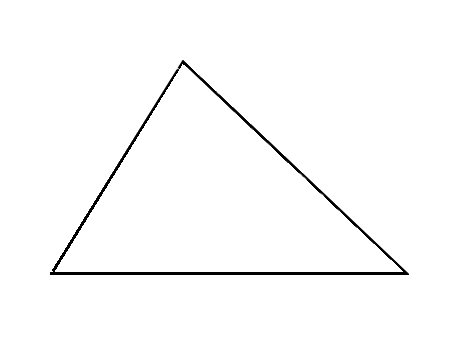Stuck on a question? Click on the solutions video below for help.

Algebra - collecting like terms

Q1

$$x =$$
(1 mark)

Q2

$$x =$$
(1 mark)

Q3

$$x =$$
(1 mark)

Q4

$$x =$$
(2 marks)

Q5

$$x =$$
(2 marks)

Q6

$$x =$$
(2 marks)

Q7$$x =$$
(2 marks)